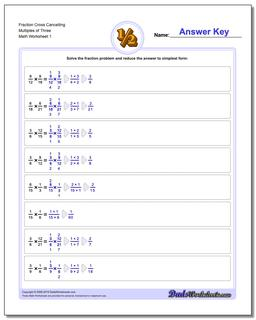# Math Worksheets: Fraction Multiplication: Fraction Multiplication: Fraction Cross Cancelling Multiples of Three## Fraction Cross Cancelling Multiples of Three

PropertyValue
DescriptionFraction Cross Cancelling Multiples of Three: The multiplying fraction worksheets in this section have a heavy emphasis on problems that require you to cross multiply (or cross cancel) to get the product.
Resource TypeWorksheet